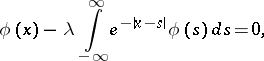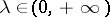Non-Fredholm integral equation

An integral equation for which some of the Fredholm theorems are false. Sometimes such an integral equation is said to be a singular integral equation.

For example, the Fourier integral equation(1)

has the solutionwhereis an arbitrary positive constant; an infinite set of linearly independent solutions corresponds to the eigen valueof (1), that is, for equation (1) Fredholm's theorem that a homogeneous equation has finitely many linearly independent solutions, does not hold.

In the case of the Lalesco–Picard integral equation(2)

everyis an eigen value, namely, to every positive numbercorrespond the two independent solutionsConsequently, for equation (2) Fredholm's theorem, that the set of eigen values of an equation is at most countable, does not hold.

The theory has been worked out in detail for two classes of non-Fredholm integral equations: equations in which the unknown function stands under the sign of an improper integral in the sense of the principal value (singular integral equations, cf. Singular integral equation); and equations in which the unknown function stands under the sign of an integral convolution transformation (cf. Integral equation of convolution type). For such equations, generally speaking, the Fredholm alternative is violated, as well as the fact that the numbers of linearly independent solutions of the homogeneous equation and its adjoint are equal.

See also the references to Integral equation of convolution type and Singular integral equation.